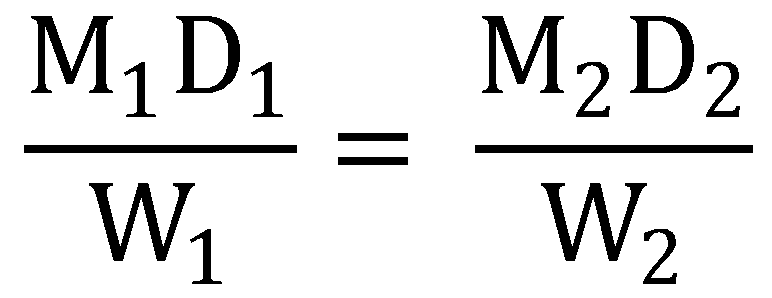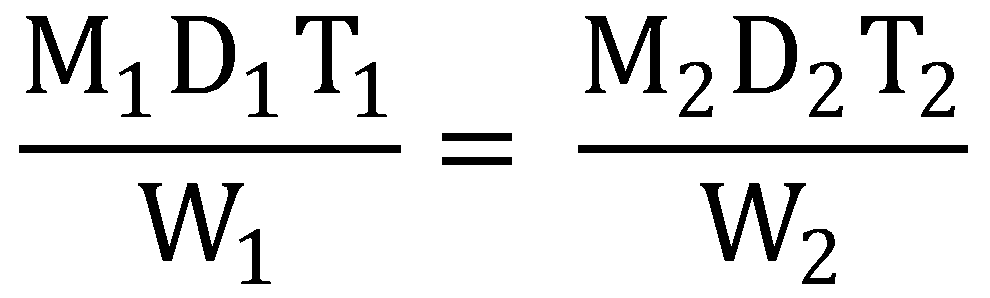Time and Work problems are most frequently asked problems in quantitative aptitude. To solve these problems very quickly, you should understand the concept of Time and Work and some shortcut methods.

If a man can do a piece of work in 5 days, then he will finish 1/5th of the work in one day.

If a man can finish 1/5th of the work in one day then he will take 5 days to complete the work.

If a man can do 5/6th of work in one hour then he will take 6/5 hours to complete the full work.

If A works three times faster than B then A takes 1/3rd the time taken by B.

Here are some shortcut rules which can be very useful while solving Time and Work problems.

In total 9 rules are given here.

Rule 1: Universal Rule
This rule can be used in almost every problems.

If M1 persons can do W1 work in D1 days and M2 persons can do W2 works in D2 days then we can sayM1D1W2 = M2D2W1

If the persons work T1 and T2 hours per day respectively then the equation gets modified toM1D1T1W2 = M2D2T2W1

If the persons has efficiency of E1 and E2 respectively then,M1D1T1E1W2 = M2D2T2E2W1

Example

5 men can prepare 10 cycles in 6 days working 6 hours a day. Then in how many days can 12 men prepare 16 cycles working 8 hours a day?
Sol:

Here M1 = 5, W1 = 10, D1 = 6, T1 = 6 and
M2 = 12, W2 = 16, T2 = 8
So from the above rule
M1D1T1W2 = M2D2T2W1
5 × 6 × 6 × 16 = 12 × D2 × 8 × 10
D2 = 3 Days.
So they will complete the work in 3 days.

Rule 2:
If A can do a piece of work in n days, then
The work done by A in one day = 1/n

Example

If A can repair 50 cycles in 5 days then A can repair 50/5 = 10 cycles in one day.

Rule 3:
If A can do a work in D1 days and B can do the same work in D2 days then A and B together can do the same workExample

If A can do a piece of work in 10 days and B can do the same work in 15 days then how long will they take if they both work together?
Sol:

A can finish the work in D1 = 10 days.
B can finish the work in D2 = 15 days.Rules 4 to 6>>